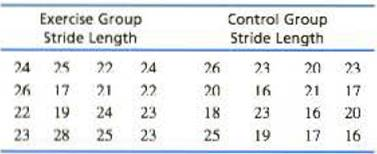Chapter 10, Problem 22PEssentials of Statistics for the B...

8th Edition
Frederick J Gravetter + 1 other
ISBN: 9781133956570

Solutions

Chapter
SectionEssentials of Statistics for the B...

8th Edition
Frederick J Gravetter + 1 other
ISBN: 9781133956570
Textbook Problem

Recent research has demonstrated that music-based physical training for elderly people can improve balance, walking efficiency, and reduce the risk of falls (Trombetti et al,. 2011). As part of the training, participants walked in time to music and responded to changes in the music’s rhythm during a one-hour per week exercise program. After six months, participants m the training group increased their walking speed and their stride length compared to individuals in the control group. The following data are similar to the results obtained in the study.Do the results indicate a significant difference in the stride length for the two groups? Use a two-tailed test with α =.05

To determine

To Find: If the results indicate significant difference in stride-length for the two groups using two-tailed test with α=0.05

Explanation

Given info:

Two samples each of size 16 is given in the question. Two-tailed hypothesis test using α=0.05 is to be performed.

Calculations:

Step 1: Null Hypothesis is H0:μ1μ2=0 and Alternate Hypothesis is H1:μ1μ20

Step 2: For the given sample, the t-statistics will have degrees of freedom equals:

df=n1+n22=16+162=30

For a two-tailed test with α=0.05 and df=30 , the critical value (CV)  is obtained from the t-table as t=±2.042

Step 3: t-statistics is calculated using formula:

t=(M1M2)(μ1μ2)sM1M2

Software procedure:

Step-by-step procedure to obtain the sample mean and standard deviations for the two groups, using the SPSS software:

• Go to Variable View> Enter the name of the variable as EXERCISE and CONTROL.
• Go to Data View>Enter the values of EXERCISE and CONTROL.
• Choose Analyze > Descriptive Statistics> choose Descriptive.
• Select EXERCISE and CONTROL and move it under variable(s)>Choose Options>Select Mean , S.E.mean, Std. Deviation and Variance
• Choose Continue> choose OK.

Output using the SPSS software is given below:

From the SPSS output, the value of two sample means and standard deviations are

M1=23M2=20s1=2.63s2=3.26

Next, calculate pooled variance, sp2 , using formula:

sp2=(df1)s12+(df2)s22df1+df2

Substitute 2.63 for s1 , 3.26 for s2 , and 15 for both df1 and df2 in the above formula

sp2=(15)2

Still sussing out bartleby?

Check out a sample textbook solution.

See a sample solution

The Solution to Your Study Problems

Bartleby provides explanations to thousands of textbook problems written by our experts, many with advanced degrees!

Get Started

Find the average value of f on [0, 8].

Single Variable Calculus: Early Transcendentals, Volume I

Domain Find the domain of the expression. 9. x21x3

Precalculus: Mathematics for Calculus (Standalone Book)

arcsin2x+arccos2x=1

Calculus: Early Transcendental Functions

14. Find .

Mathematical Applications for the Management, Life, and Social Sciences

For , f′(x) =

Study Guide for Stewart's Single Variable Calculus: Early Transcendentals, 8th